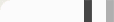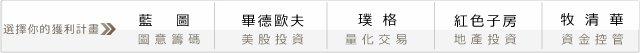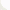## 開發商品的交易系統 - 基礎篇N日移動平均線＝N日收市價之和／Ninput:ExitType(2) ;
vars: IsBalanceDay(False),MP(0),PF(0),PL(0);

inputs:Ratio(16),LongLenA(8),ShortLenA(4),LongLenB(2),ShortLenB(5);
Vars:Price(AvgPrice),Base(1),HighLine(0),LowLine(0),MedLine(0),SumLine(0) ;

MP = MarketPosition ;
if DAYofMonth(Date) > 14 and DAYofMonth(Date) < 22 and DAYofWeek(Date)= 3 then isBalanceDay = True else isBalanceDay =False ;

{ 高低點初值設定 }
HighLine = Average(Price,Ratio) ;
LowLine = Average(Price,Ratio) ;

{ 連續期間的均線高低點與中值計算 }

SumLine = 0 ;
for Base = Ratio to 20+Ratio Begin
if Average(Price,Base) > HighLine then HighLine = Average(Price,Base) ;
if Average(Price,Base) < LowLine then LowLine = Average(Price,Base) ;
SumLine = SumLine+Average(Price,Base) ;
end;
MedLine = SumLine/20 ;

{ 買方環境建立 }

Condition1 = Close < MedLine or Close < HighLine ;

{ 通道下緣上升 } Condition2 = LowLine > LowLine and LowLine > LowLine ;

{ 賣方環境建立 }
Condition3 = Close > MedLine or Close > HighLine ;
{ 通道上緣下降 }
Condition4 = HighLine > HighLine and HighLine > HighLine;

{ 通道寬度 }
HLRange = (HighLine-LowLine) ;

{ 空手進場 規則 }
{ 買方環境 + 通道壓縮 }if MP = 0 and HLRange = Lowest(HLRange,LongLenA) and Condition1 and Condition2 then Buy next bar at HighLine stop ;
{賣方環境 + 通道壓縮 }
if MP = 0 and HLRange = Lowest(HLRange,ShortLenA) and Condition3 and Condition4 then Sell next bar at LowLine Stop ;

{ 手中有部位反手單 + 通道擴張 }
if MP < 0 and HLRange = Highest(HLRange,LongLenB) then Buy next bar at HighLine stop ;
if MP > 0 and HLRange = Highest(HLRange,ShortLenB) then Sell next bar at LowLine Stop ;

{ 一般出場 }
if ExitType = 1 then SetStopLoss(PL * BigPointValue) ;

if ExitType = 2 then Begin
SetStopLoss(PL * BigPointValue) ;
setProfitTarget(PF * BigPointValue) ;
end;

if ExitType = 3 then Begin
if MP > 0 and BarsSinceEntry = NBarL then ExitLong next bar at Market ;
if MP < 0 and BarsSinceEntry = NBarS then ExitShort next bar at Market ; end; if ExitType = 4 then Begin SetStopLoss(PL * BigPointValue) ; setProfitTarget(PF * BigPointValue) ; if MP > 0 and BarsSinceEntry = NBarL then {Sell } ExitLong next bar at Market ;
if MP < 0 and BarsSinceEntry = NBarS then {Buy} ExitShort next bar at Market ;
end;

if IsBalanceDay then setExitonClose ;#### 0 意見: